Courses

# NCERT Solutions(Part- 5)- Linear Equations in One Variable Class 8 Notes | EduRev

## Mathematics (Maths) Class 8

Created by: Vp Classes

## Class 8 : NCERT Solutions(Part- 5)- Linear Equations in One Variable Class 8 Notes | EduRev

The document NCERT Solutions(Part- 5)- Linear Equations in One Variable Class 8 Notes | EduRev is a part of the Class 8 Course Mathematics (Maths) Class 8.
All you need of Class 8 at this link: Class 8

EXERCISE 2.5
Question 1: Solve the following linear equations.

1.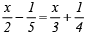2.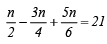3.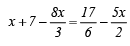4.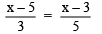5.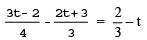6.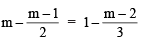Ans:
1.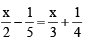Transposing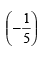to RHS and x/3  to LHS, we have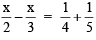or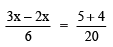or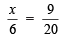or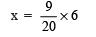(Multiplying both sides by 6)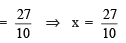Check: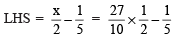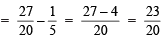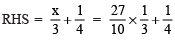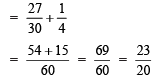∴ LHS = RHS

2.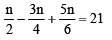∵  LCM of 2, 4 and 6 = 12

∴  Multiplying both sides by 12, we have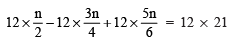or

6n – 9n + 10n = 252

or

7n = 252

or

n = 252/7 = 36

∴  n = 36

Check: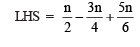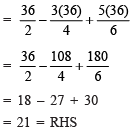∴ LHS = RHS

3.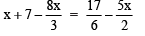∵ LCM of 3, 6 and 2 is 6.

∴ Multiplying both sides by 6, we have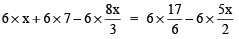or 6x + 42 – 16x = 17 – 15x

or (6 – 16)x + 42 = 17 – 15x

or –10x + 42 = 17 – 15x

Transposing 42 to RHS and –15x to LHS, we have

–10x + 15x = 17 – 42 or 5x = –25

or

5x = –25

or

x = -25/5 = -5    (Dividing both sides by 5)

∴ x  = –5

Check: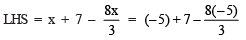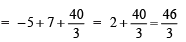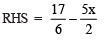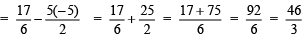∴ LHS = RHS

4.∵ LCM of 3 and 5 is 15.

∴ Multiplying both sides by 15, we have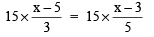or

5(x – 5) = 3(x – 3)

or

5x – 25 = 3x – 9

Transposing (–25) to RHS and 3x to LHS, we have
5x – 3x = –9 + 25

or

2x = 16

or

x = 16/2   (Dividing both sides by 2)

∴ x = 8

Check: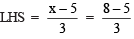= 3/3 = 1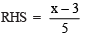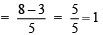LHS = RHS

5.∵  LCM of 4 and 3 is 12.

∴  Multiplying both sides by 12, we have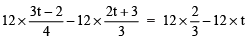or

3(3t – 2) – 4(2t + 3) = (4 x2) – 12t
or

9t – 6 – 8t – 12 = 8 – 12t
or

(9 – 8)t – (6 + 12) = 8 – 12t

or

t – 18 = 8 – 12t

Transposing –18 to RHS and –12t to LHS, we have
t + 12t = 8 + 18

or

13t = 26

or

t = 26/13

∴ t = 2

Check: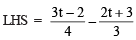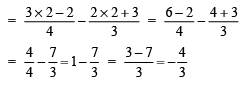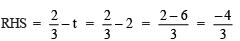LHS = RHS

6.Since, LCM of 2 and 3 is 6.

∴   Multiplying both sides by 6, we have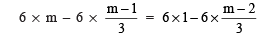or

6m – 3(m – 1) = 6 – 2(m – 2)
or

6m – 3m + 3 = 6 – 2m + 4
or

(6 – 3)m + 3 = (6 + 4) – 2m
or

3m + 3 = 10 – 2m
Transposing 3 to RHS and –2m to LHS, we have
3m + 2m = 10 – 3

or  5m = 7

or

m = 7/5    (Dividing both sides by 5)

Check: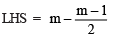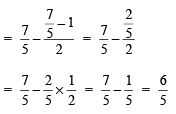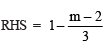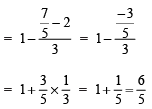LHS = RHS

Question 2: Simplify and solve the following linear equations.
7 . 3(t – 3) = 5(2t + 1)
8. 15(y – 4) – 2(y – 9) + 5(y + 6) = 0
9. 3(5z – 7) – 2(9z – 11) = 4(8z – 13) – 17
10. 0.25(4f – 3) = 0.05(10f – 9)

Ans: 7. 3(t – 3) = 5(2t + 1)
or

3t – 9 = 10t + 5

Transposing (–9) to RHS and 10t to LHS, we have
3t – 10t = 5 + 9
or

–7t = 14

or
t = 14/-7 = -2               (Dividing both sides by –7)

∴  t = –2

8. 15(y – 4) – 2(y – 9) + 5(y + 6) = 0

Opening the brackets, we have

15y – 60 – 2y + 18 + 5y + 30 = 0

Collecting the like terms,
(15 – 2 + 5)y + (–60 + 18 + 30) = 0
or

18y + (–12) = 0

Transposing (–12) to RHS, we have

18y = 12

or

y = 12/18 = 2/3  (Dividing both sides by 18)

∴  y = 2/3

9. 3(5z – 7) – 2(9z – 11) = 4(8z – 13) – 17

Opening the brackets, we have
15z – 21 – 18z + 22 = 32z – 52 – 17
Collecting the like terms on both sides,
(15 – 18)z + (–21 + 22) = 32z + (–52 – 17)

or

–3z + 1 = 32z – 69
Transposing 1 to RHS and 32z to LHS, we have
–3z – 32z = –69 – 1

or

–35z = –70

or   z = -70/-35 = 2   (Dividing both sides by –35)

∴  z = 2

10. 0.25(4f – 3) = 0.05(10f – 9)

Opening the brackets, we have

0.25 * 4f – 3 * 0.25 = 0.05 *10f – 0.05 * 9
or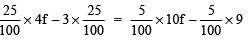or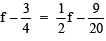Transposing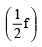to RHS and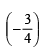to LHS, we have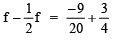or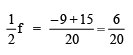or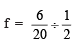(Dividing both sides by 1/2)

or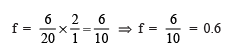## Mathematics (Maths) Class 8

212 videos|147 docs|48 tests

,

,

,

,

,

,

,

,

,

,

,

,

,

,

,

,

,

,

,

,

,

;